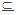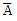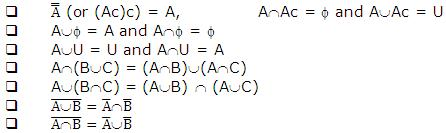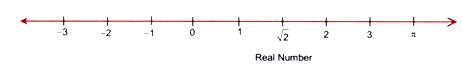Click to Chat

1800-1023-196

+91-120-4616500

CART 0

• 0

MY CART (5)

Use Coupon: CART20 and get 20% off on all online Study Material

ITEM
DETAILS
MRP
DISCOUNT
FINAL PRICE
Total Price: Rs.

There are no items in this cart.
Continue Shopping```Set Theory

Table of Content

Set

Union of Sets

Intersection of Sets

Difference of two Sets

Subset of a Set

Equality of two Sets

Natural Numbers

Rational Numbers

Irrational Numbers

Real Numbers

Intervals

SetA set is a well-defined collection of objects or elements. Each element in a set is unique. Usually but not necessarily a set is denoted by a capital letter e.g., A, B, ....., U, V etc. and the elements are enclosed between brackets { }, denoted by small letters a, b, ....., x, y etc. For example:

A = Set of all small English alphabets
= {a, b, c, ....., x, y, z}
B = Set of all positive integers less than or equal to 10
= {1, 2, 3, 4, 5, 6, 7, 8, 9, 10}
R  = Set of real numbers
= {x : -  ∞ < x <  ∞}
The elements of a set can be discrete (e.g. set of all English alphabets) or continuous (e.g. set of real numbers). The set may contain finite or infinite number of elements. A set may contain no elements and such a set is called Void set or Null set or empty set and is denoted by Φ(phi). The number of elements of a set A is denoted as n(A) and hence n(Φ) = 0 as it contains no element.

Union of Sets

Union of two or more sets is the best of all elements that belong to any of these sets. The symbol used for union of sets is 'U'
i.e. AUB = Union of set A and set B
= {x : x ε A or x ε B (or both)}
e.g. If A = {1, 2, 3, 4} and B = {2, 4, 5, 6} and C = {1, 2, 6, 8} then AUBUC = {1, 2, 3, 4, 5, 6 8}.

Intersection of Sets

It is the set of all of the elements, which are common to all the sets. The symbol used for intersection of sets is '∩'.

i.e. A∩B = {x : x ε A and x ε B}
e.g. If A = {1, 2, 3, 4} an B = {2, 4, 5, 6} and C = {1, 2, 6, 8}, then A∩B∩C = {2}.
Remember that n(AUB) = n(A) + n(B) -(A∩B).

Difference of two Sets

The difference of set A to B denoted as A - B is the set of those elements that are in the set A but not in the set B i.e. A - B = {x : x ε A and xA}.
In general A-B≠B-A
e.g. If A = {a, b, c, d} and B = {b, c, d} then A - B = {a, d} and B - A = {e, f}.

Subset of a Set

A set A is said to be a subset of the set B is each element of the set A is also the element of the set B. The symbol used is ''  i.e. AB <=> (x ε A => x ε B).
Each set is a subset of its own set. Also a void set is a subset of any set. If there is at least one element in B which does not belong to the set A, then A is a proper subset of set B and is denoted as AB.
e.g. If A = {a, b, c, d} and B = {b, c, d} then B   A or equivalently AB (i.e. A is a super set of B).

Equality of two Sets

Sets A and B are said to be equal if AB and BA and we write A = B.

Universal Set

As the name implies, it is a set with collection of all the elements and is usually denoted by U. e.g. set of real numbers R is a universal set whereas a set A = [x : x < 3} is not a universal set as it does not contain the set of real numbers x > 3. Once the universal set is known, one can define the Complementary set of a set as the set of all the elements of the universal set which do not belong to that set. e.g. If A = {x : x < 3 then(or Ac) = complimentary set of A = {x : x > 3}. Hence we can say that A U= U i.e. Union of a set and its complimentary is always the Universal set and A ∩= f i.e. intersection of the set and its complimentary is always a void set. Some of the useful properties of operation on sets are as follows:Illustration:

If A = {a, b, c} and B = {b, c, d} then evaluate A υ B, A ∩ B, A - B and  B - A.

Solution:
A U B = {x : x ε A or x ε B} = {a, b, c, d}
A ∩ B = {x : x ε A or x ε B} = {b, c}
A - B = {x : x ε A and xB} = {a}
B - A = {x : x ε B and xA} = {d}

Natural Numbers

The numbers 1, 2, 3, 4 ......... are called natural numbers, their set is denoted by N.
Thus N = {1, 2, 3, 4, 5 ......}

Integers

The numbers .....-3, -2, -1, 0, 1, 2, 3 ....... are called integers and the set is denoted by I or Z.
Thus I (or Z) = {.... -3, -2, -1, 0, 1, 2, 3 ....}. Including among set of integers are:

Set of positive integers denoted by 1+ and consists of {1, 2, 3,....} (≡N)

Set of negative integers, denoted by 1- and consists of {..., 3, -2, -1}

Set of non-negative integers {0, 1, 2,...} called as set of whole numbers

Set of non-positive integers {..., -3, -2, -1, 0}

Rational Numbers

All numbers of the form p/q where p and q are integers and q ≠ 0, are called rational numbers and their set is denoted by Q.
Thus Q = {p/q : p,q ε I and q≠0 and HCF of p,q, is 1}. It may be noted that every integer is a rational number since it can be written as p/1. It may also be noted that all recurring decimals are rational numbers. e.g., p = 0.3 = 0.33333.....
And 10p - p = 3 => 9p = 3 => p = 3/9 => p = 1/3, which is a rational number.

Irrational Numbers

There are numbers which cannot be expressed in p/q form. These numbers are called irrational numbers and their set is denoted by Qc (i.e. complementary set of Q) e.g. √2, 1 + √3, p etc. Irrational numbers cannot be expressed as recurring decimals.

Real Numbers

The complete set of rational and irrational numbers is the set of real numbers and is denoted by R. Thus R = Q υ Qc.
It may be noted that NIQR. The real numbers can also be expressed in terms of position of a point on the real line. The real line is the number line where the position of a point relative to the origin (i.e. 0) represents a unique real number and vice versa.All the numbers defined so far follow the order property i.e. if there are two numbers a and b then either a < b or a = b or a > b.

Intervals

Intervals are basically subsets of R and are of very much importance in calculus as you will get to know shortly. If there are two numbers a, b ε R such that a < b, we can define four types of intervals as follows:

Open interval: (a, b) = {x : a < x < b} i.e. end points are not included

Closed interval: [a, b] = {x : a < x < b} i.e. end points are also included.

This is possible only when both a and b are are finite.

Open-closed interval : (a, b] = {x : a < x < b}

Closed-open interval : [a, b) = {x : a < x < b}

The infinite intervals are defined as follows:

(a, ∞) = {x : x > a}

[a, ∞) = {x : x > a}

(-∞, b) = {x : x < b)

(-∞, b] = {x : x < b}

Intervals are particularly important in solving inequalities or in finding domains etc.
Free study material for the preparation of IIT JEE, AIEEE and other engineering examinations is available online at askIITians.com. Study Physics, Chemistry and Mathematics at askIITians website and be a winner. We offer numerous live online courses as well for live online IIT JEE preparation - you do not need to travel anywhere any longer - just sit at your home and study for IIT JEE live online with askIITians.com

To read more, Buy study materials of Set Relations and Functions comprising study notes, revision notes, video lectures, previous year solved questions etc. Also browse for more study materials on Mathematics here.
```### Course Features

• 731 Video Lectures
• Revision Notes
• Previous Year Papers
• Mind Map
• Study Planner
• NCERT Solutions
• Discussion Forum
• Test paper with Video Solution